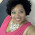## Monday, 28 October 2013

### Logistic Distribution CDF and Quantile Calculator

An implementation of the Logistic Distribution CDF and Quantile function Calculator occurs below. The Logistic distribution function (for location $\mu$ real and scale $s>0$) is:-

$\Large \frac{exp(-\frac{(x-\mu)}{s})}{s(1+exp(-\frac{(x-\mu)}{s}))^2}$

where the random variable is $-\infty < x < \infty$. The location and scale fields have to be filled in, as well as two out of the three fields which are labelled Lower Limit, Upper Limit and Probability. The lower limit field needs to contain a real number greater or the string -inf (for minus infinity). The upper limit field needs to contain either a number greater than or equal to 0 or the string inf (for plus infinity). The probability field must contain a number only.

 $\mu$: s:

 Lower limit: Upper limit: Probablility:

 Plot of distribution ($f(x)$) values against $x$ values $f(x)$ $x$

1.2.